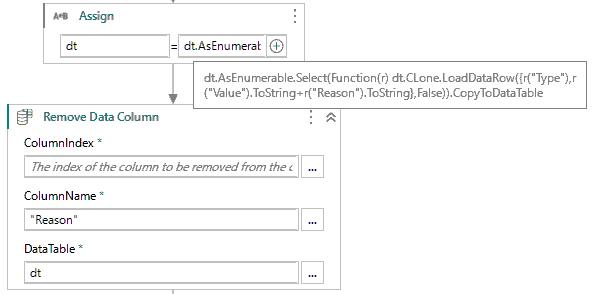# How to combine two column

I need to combine two column

Type. Value. Reason…

A. Amount is 20.1 amount is valid

Output should be:

Type. Value

A. Amount is 20.1 amount is valid

Hi,

Can you try the following?``````dt = dt.AsEnumerable.Select(Function(r) dt.CLone.LoadDataRow({r("Type"),r("Value").ToString+r("Reason").ToString},False)).CopyToDataTable
``````

Regards,